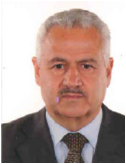# College of Science

## Calculus I

 Course Code C0499 Th 3 Pr 3 CrHrs 3

#### LecturerAbdulkader Ahmad Hamy Lecturer

Overview and reference for the mathematical techniques and calculus course. Some topics may be familiar while other may not. Depending on the mathematical level of the course. Each topic is covered in sufficient.

1. The purpose of this course is to present basic calculus concepts and show how to deal with problems.
2. In this way the development of the calculus comes about as a means of solving problems.
3. The aim of this course is to provide you with review and reference for the mathematical techniques you will need in your calculus course. Some topics may be familiar to you while other may not. Depending on the mathematical level of your course .Each topics is covered in sufficient

4. Depth to allow you to perform the mathematical manipulations necessary for a particular problem. The explanation is, of necessity, and brief.

 Distribution of Mark Final Mark Final Exam Second Term Mid-Year First Term 100 Prac. Theor. Prac. Theor. Prac. Theor. Prac. Theor. - 60 - - - 30 - 10
 References SN George B. Thomas, Jr. " Calculus", International Edition 11'th, Addison-Wesley, 2005. 1 Ross L. Fenny and George B. Thomas, Jr. " Calculus", Addison-Wesley, 1990. 2 Frank E. Junior and Fillip Schmidt, "Collage Mathematics", Shum's Series, McGraw-Hill Inc. 1992, Academia International 2001. 3
 Subject Week Introduction First Functions Second Domain function Third Test function Fourth absolute Fifth Value function Sixth Mathematical Models. Seventh Matrices Fundamentals Eighth Matrices Introduction, Ninth Matrix Operating (+, –, ÷, ×, . ) Tenth Basics of Statistic Eleventh